## Introduction

We look at some of the basic operations associated with probability distributions. There are a large number of probability distributions available, but we only look at a few. If you would like to know what distributions are available you can do a search using the command help.search(“distribution”).

Here we give details about the commands associated with the normal distribution and briefly mention the commands for other distributions. The functions for different distributions are very similar where the differences are noted below.

For every distribution there are four commands. The commands for each distribution are prepended with a letter to indicate the functionality:

• “d” returns the height of the probability density function
• “p” returns the cumulative density function
• “q” returns the inverse cumulative density function (quantiles)
• “r” returns randomly generated numbers

## The Normal Distribution

There are four functions that can be used to generate the values associated with the normal distribution.

### dnorm

The first function we look at it is `dnorm`. Given a set of values it returns the height of the probability distribution at each point. If you only give the points it assumes you want to use a mean of zero and standard deviation of one. There are options to use different values for the mean and standard deviation, though:

``dnorm(0)``
``##  0.3989423``
``dnorm(0) * sqrt(2 * pi)``
``##  1``
``dnorm(0, mean = 4)``
``##  0.0001338302``
``dnorm(0, mean = 4, sd = 10)``
``##  0.03682701``
``````v <- c(0, 1, 2)
dnorm(v)``````
``##  0.39894228 0.24197072 0.05399097``
``````x <- seq(-20, 20, by = .1)
y <- dnorm(x)
plot(x, y)``````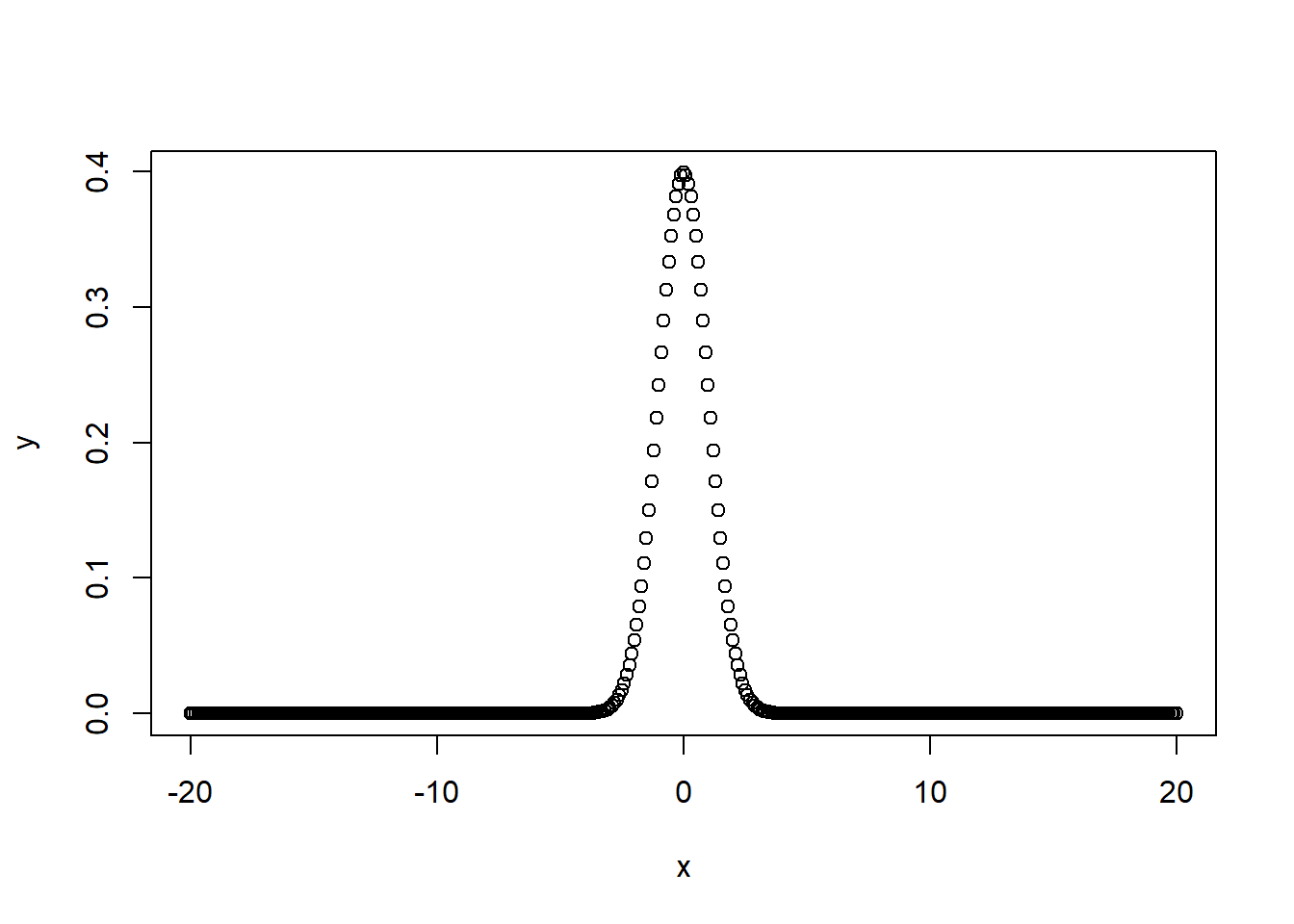``````y <- dnorm(x, mean = 2.5, sd = 0.1)
plot(x, y)``````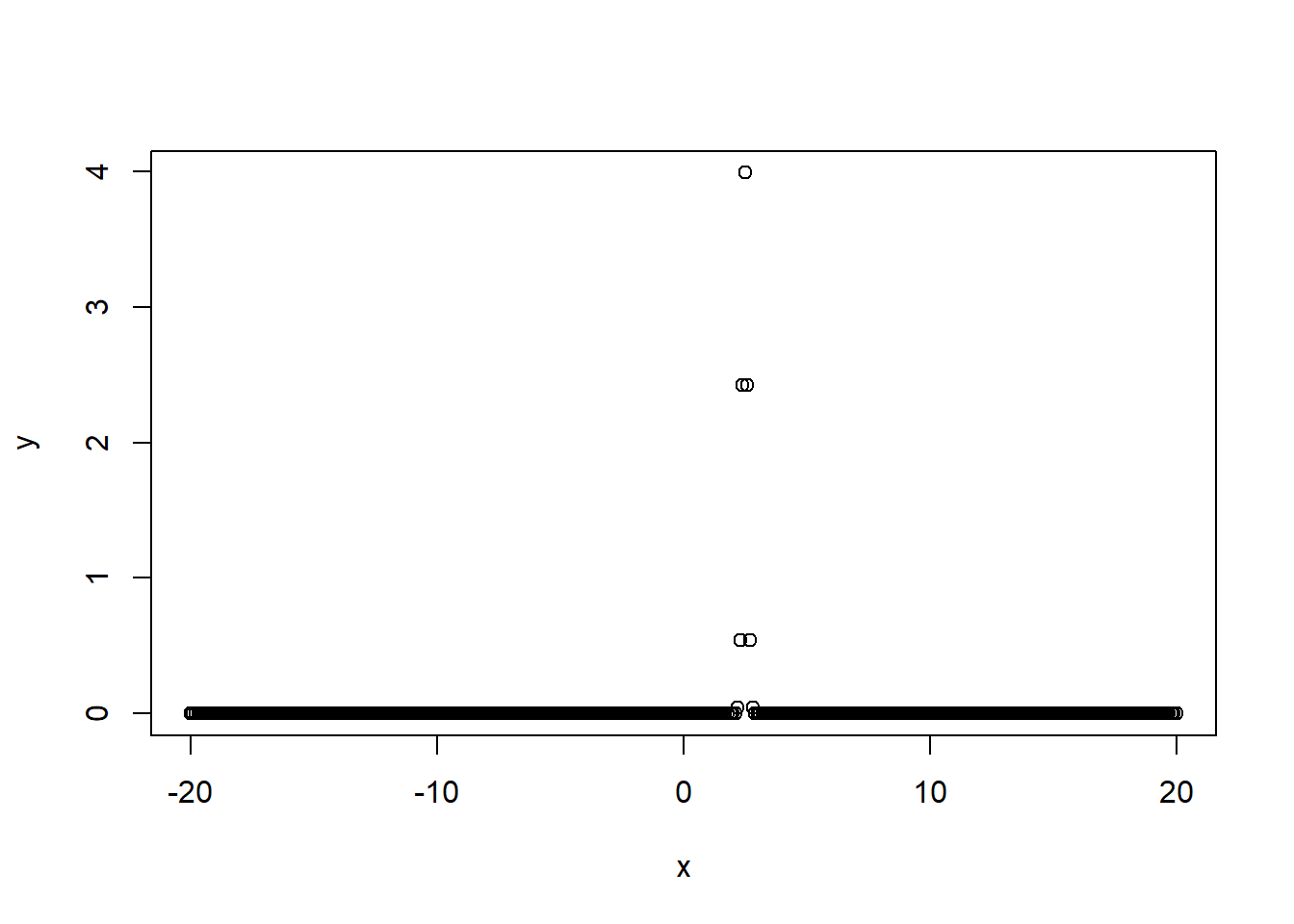### pnorm

The second function we examine is `pnorm`. Given a number or a list it computes the probability that a normally distributed random number will be less than that number. This function also goes by the rather ominous title of the “Cumulative Distribution Function.” It accepts the same options as dnorm:

``pnorm(0)``
``##  0.5``
``pnorm(1)``
``##  0.8413447``
``pnorm(0, mean = 2)``
``##  0.02275013``
``pnorm(0, mean = 2, sd = 3)``
``##  0.2524925``
``````v <- c(0, 1, 2)
pnorm(v)``````
``##  0.5000000 0.8413447 0.9772499``
``````x <- seq(-20, 20, by = .1)
y <- pnorm(x)
plot(x,y)``````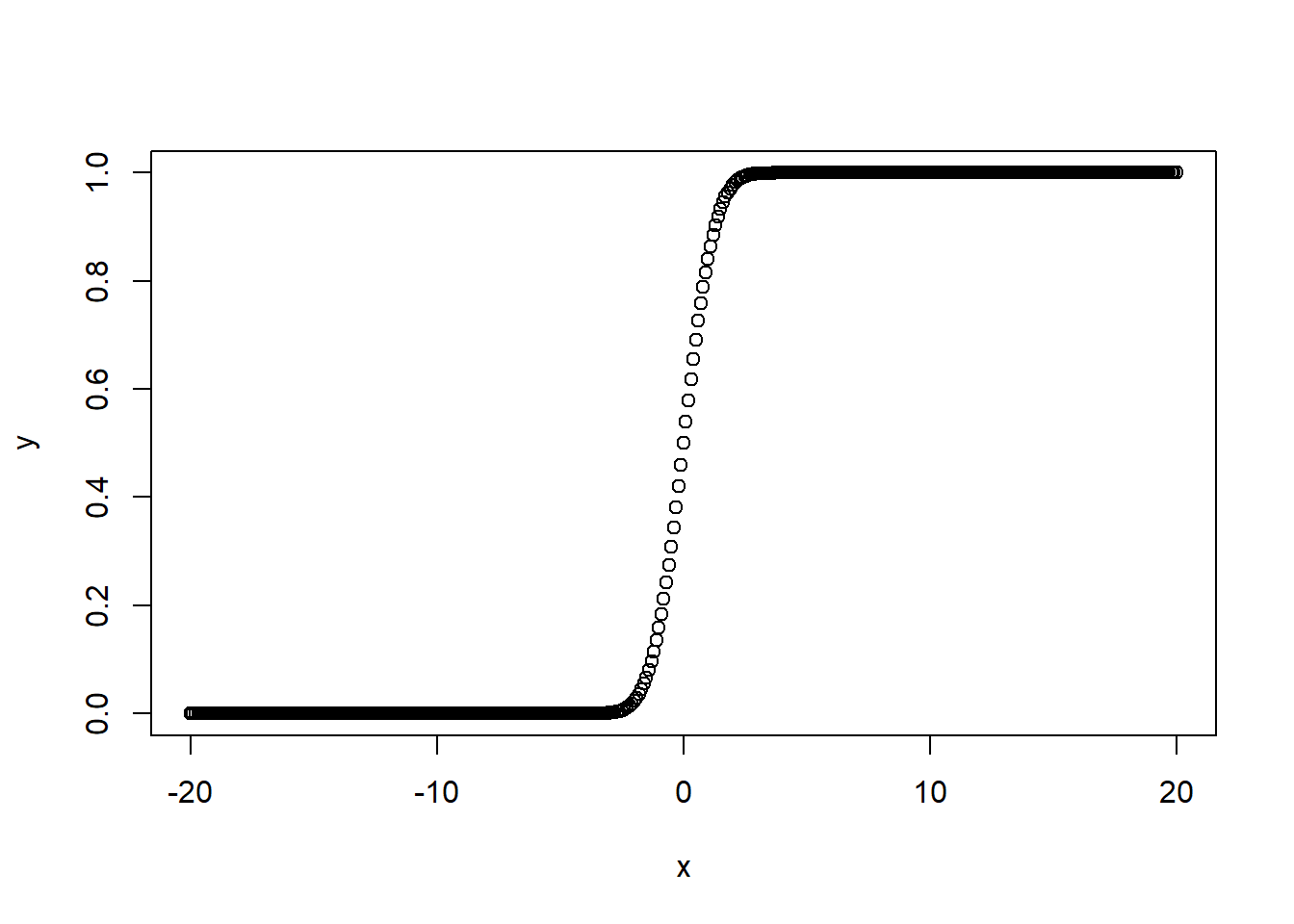``````y <- pnorm(x, mean = 3, sd = 4)
plot(x,y)``````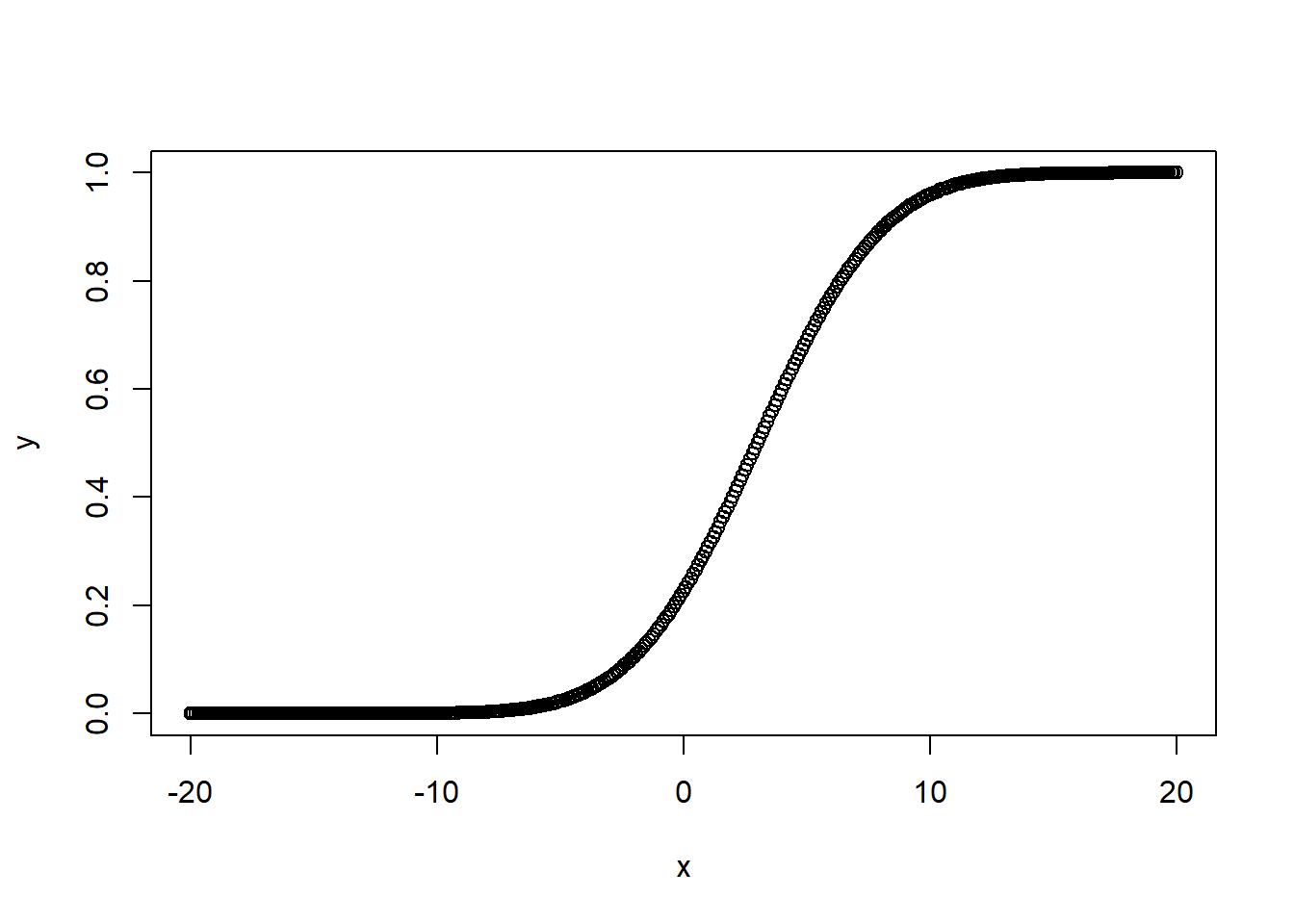If you wish to find the probability that a number is larger than the given number you can use the lower.tail option:

``pnorm(0, lower.tail = FALSE)``
``##  0.5``
``pnorm(1, lower.tail = FALSE)``
``##  0.1586553``
``pnorm(0, mean = 2, lower.tail = FALSE)``
``##  0.9772499``

### qnorm

The next function we look at is `qnorm` which is the inverse of pnorm. The idea behind qnorm is that you give it a probability and it returns the number whose cumulative distribution matches the probability. For example, if you have a normally distributed random variable with mean zero and standard deviation one, then if you give the function a probability it returns the associated Z-score:

``qnorm(0.5)``
``##  0``
``qnorm(0.5, mean = 1)``
``##  1``
``qnorm(0.5, mean = 1, sd = 2)``
``##  1``
``qnorm(0.5, mean = 2, sd = 2)``
``##  2``
``qnorm(0.5, mean = 2, sd = 4)``
``##  2``
``qnorm(0.25, mean = 2, sd = 2)``
``##  0.6510205``
``qnorm(0.333)``
``##  -0.4316442``
``qnorm(0.333, sd = 3)``
``##  -1.294933``
``qnorm(0.75, mean = 5, sd = 2)``
``##  6.34898``
``````v = c(0.1, 0.3, 0.75)
qnorm(v)``````
``##  -1.2815516 -0.5244005  0.6744898``
``````x <- seq(0, 1, by = .05)
y <- qnorm(x)
plot(x, y)``````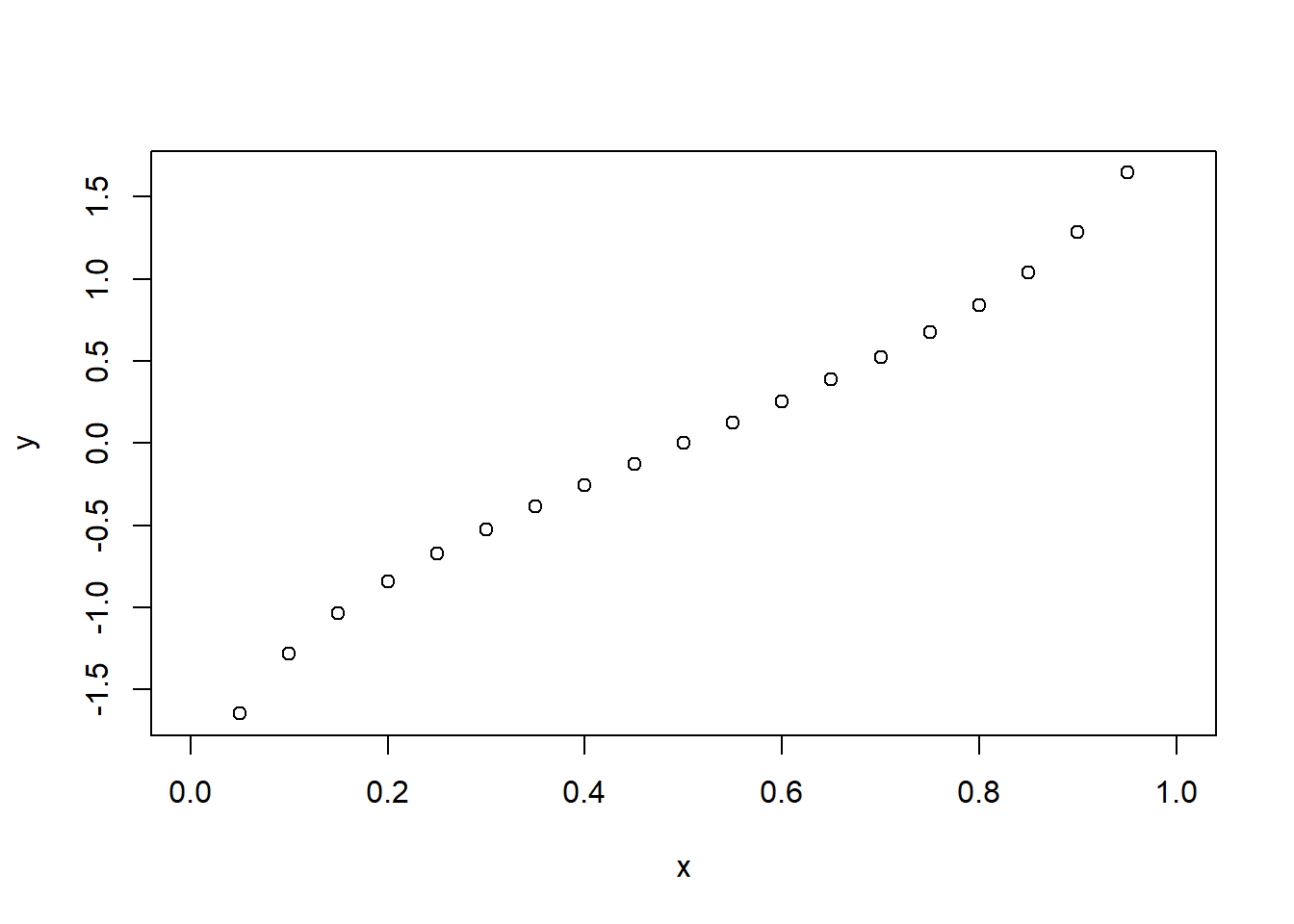``````y <- qnorm(x, mean = 3, sd = 2)
plot(x, y)``````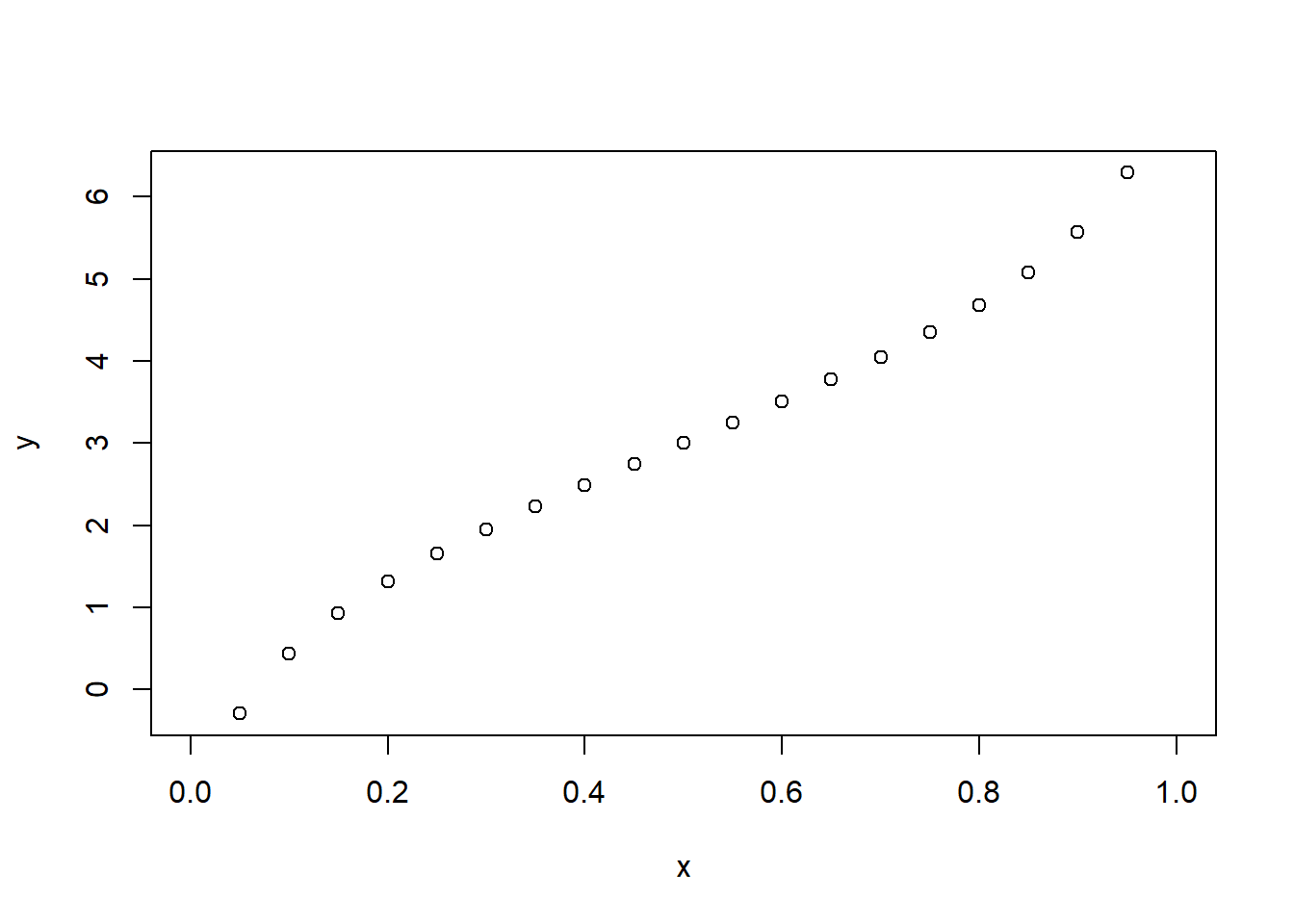``````y <- qnorm(x, mean = 3, sd = 0.1)
plot(x, y)``````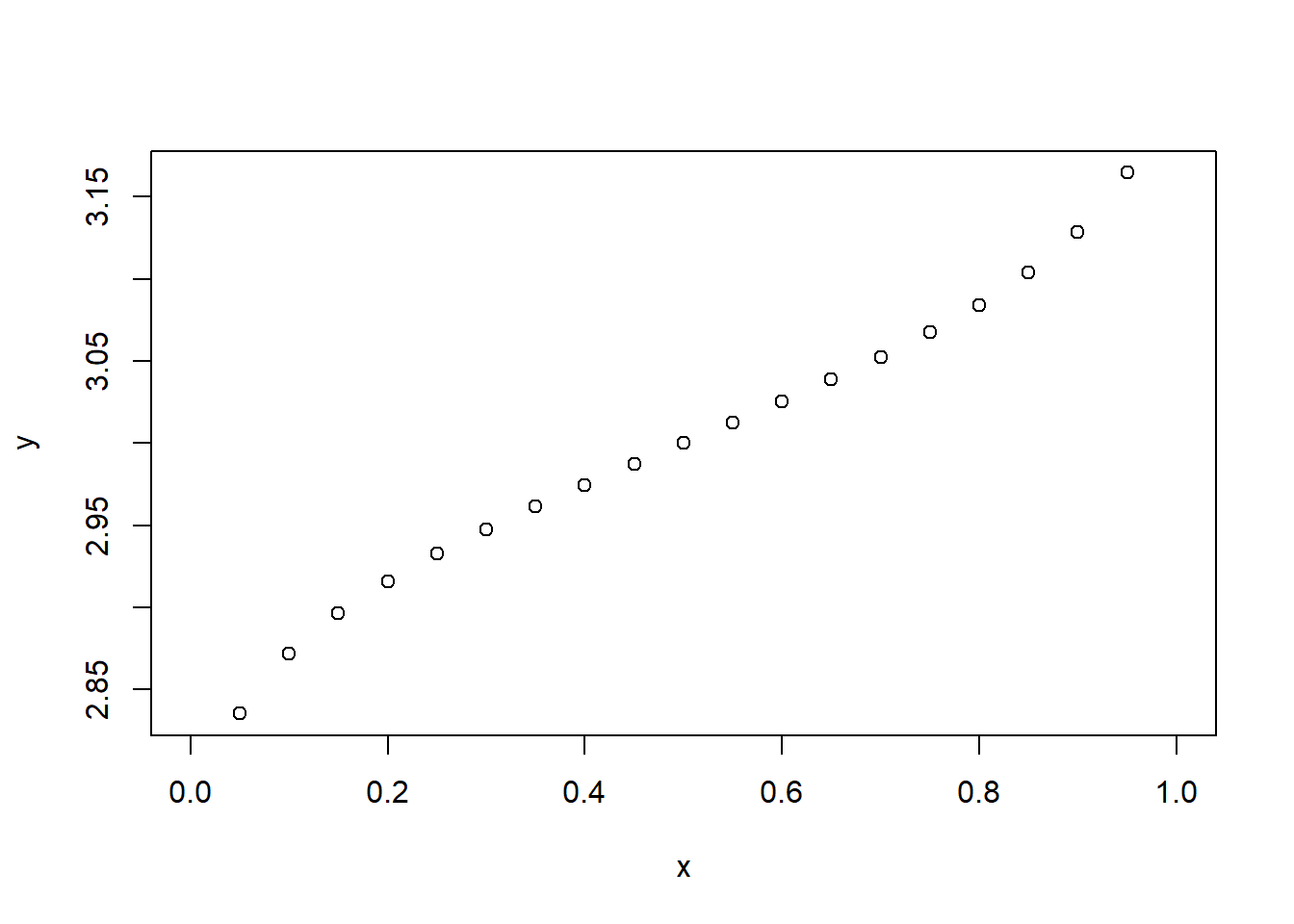### rnorm

The last function we examine is the `rnorm` function which can generate random numbers whose distribution is normal. The argument that you give it is the number of random numbers that you want, and it has optional arguments to specify the mean and standard deviation:

``rnorm(4)``
``##   0.07298852 -1.32810844 -0.28272296  0.12861971``
``rnorm(4, mean = 3)``
``##  3.493688 2.070186 2.933694 2.899632``
``rnorm(4, mean = 3, sd = 3)``
``##  1.929643 3.092662 2.095764 6.711923``
``rnorm(4, mean = 3, sd = 3)``
``##  3.837437 3.038792 3.543452 4.120511``
``````y <- rnorm(200)
hist(y)``````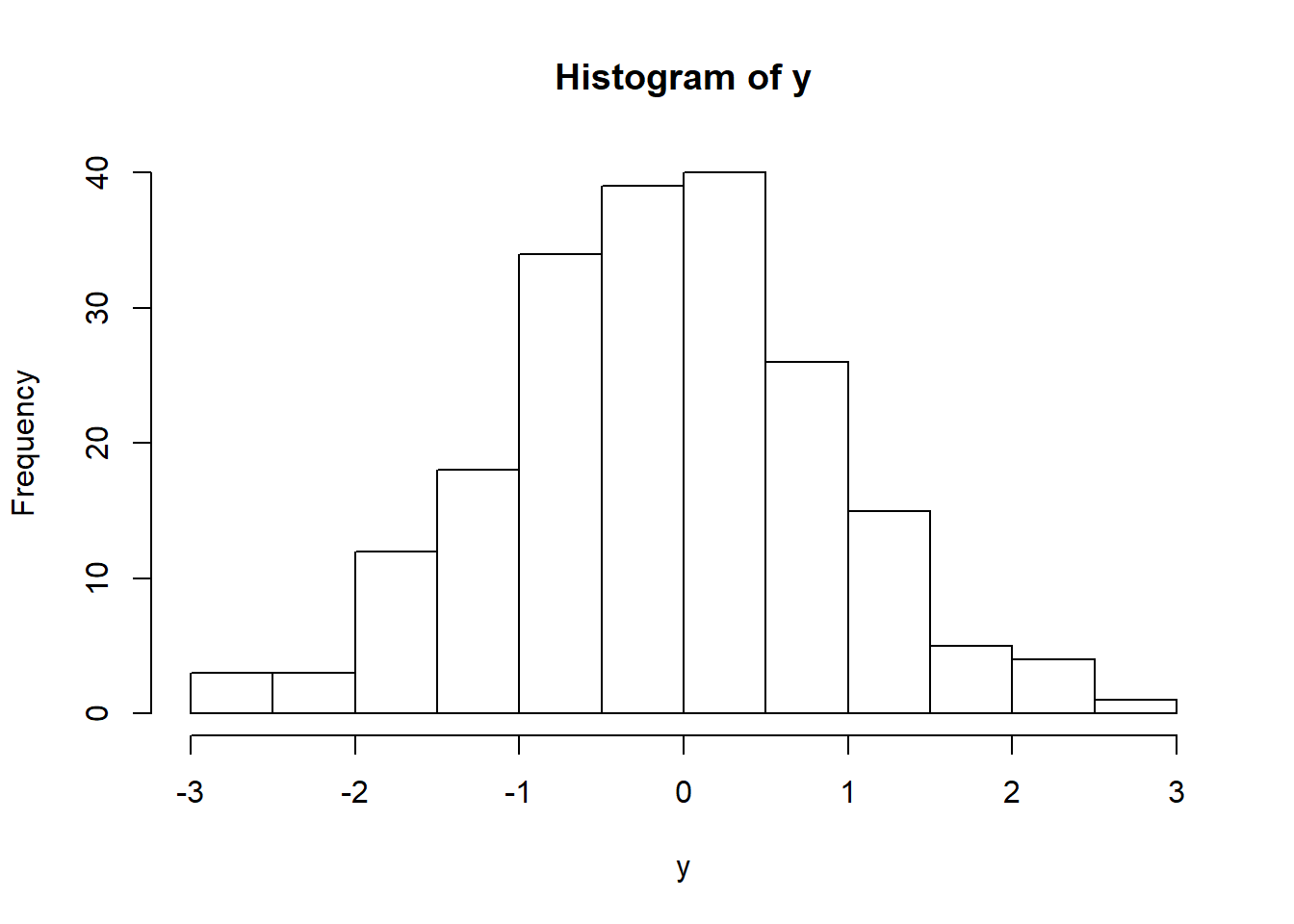``````y <- rnorm(200, mean = -2)
hist(y)``````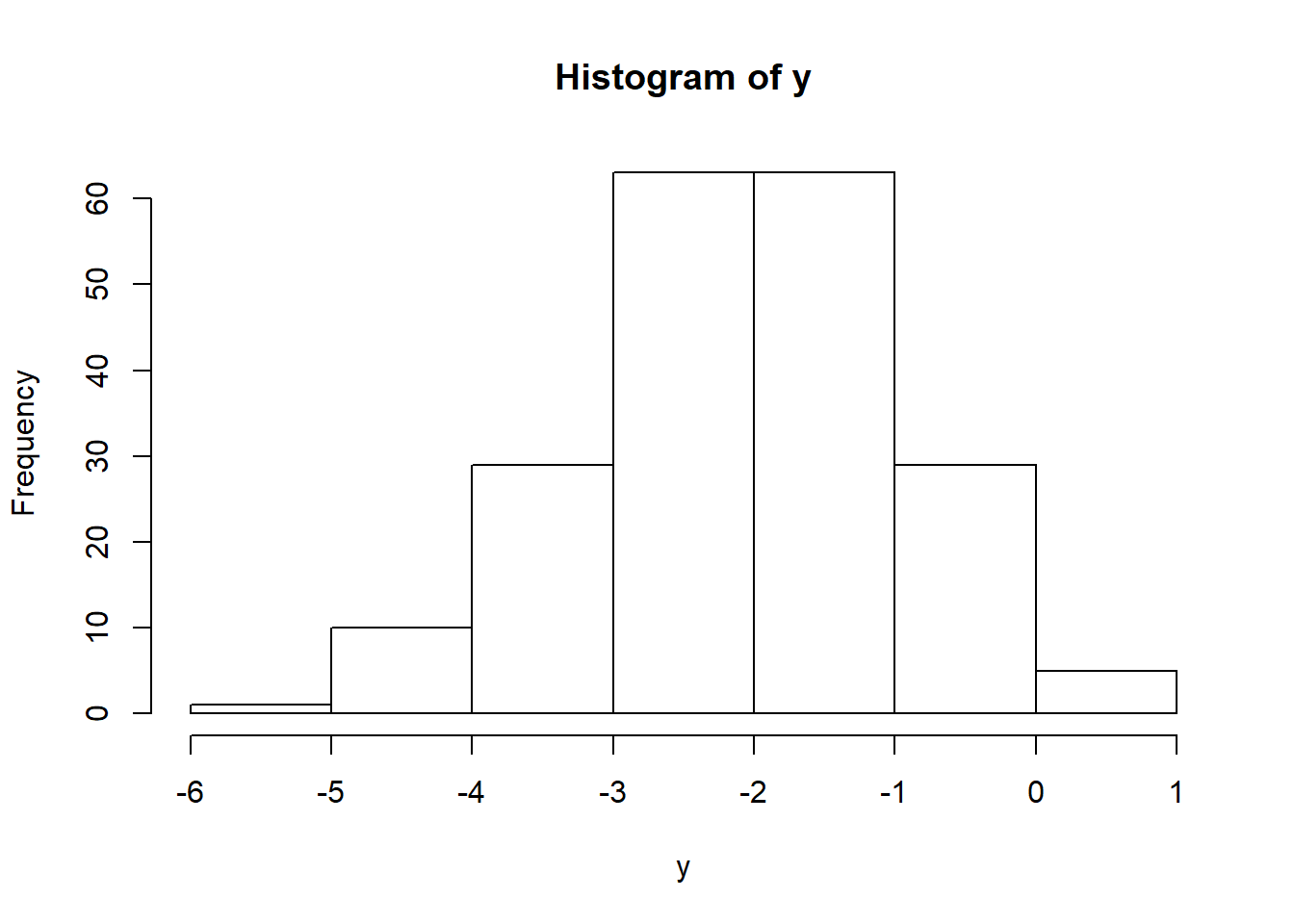``````y <- rnorm(200, mean = -2, sd = 4)
hist(y)``````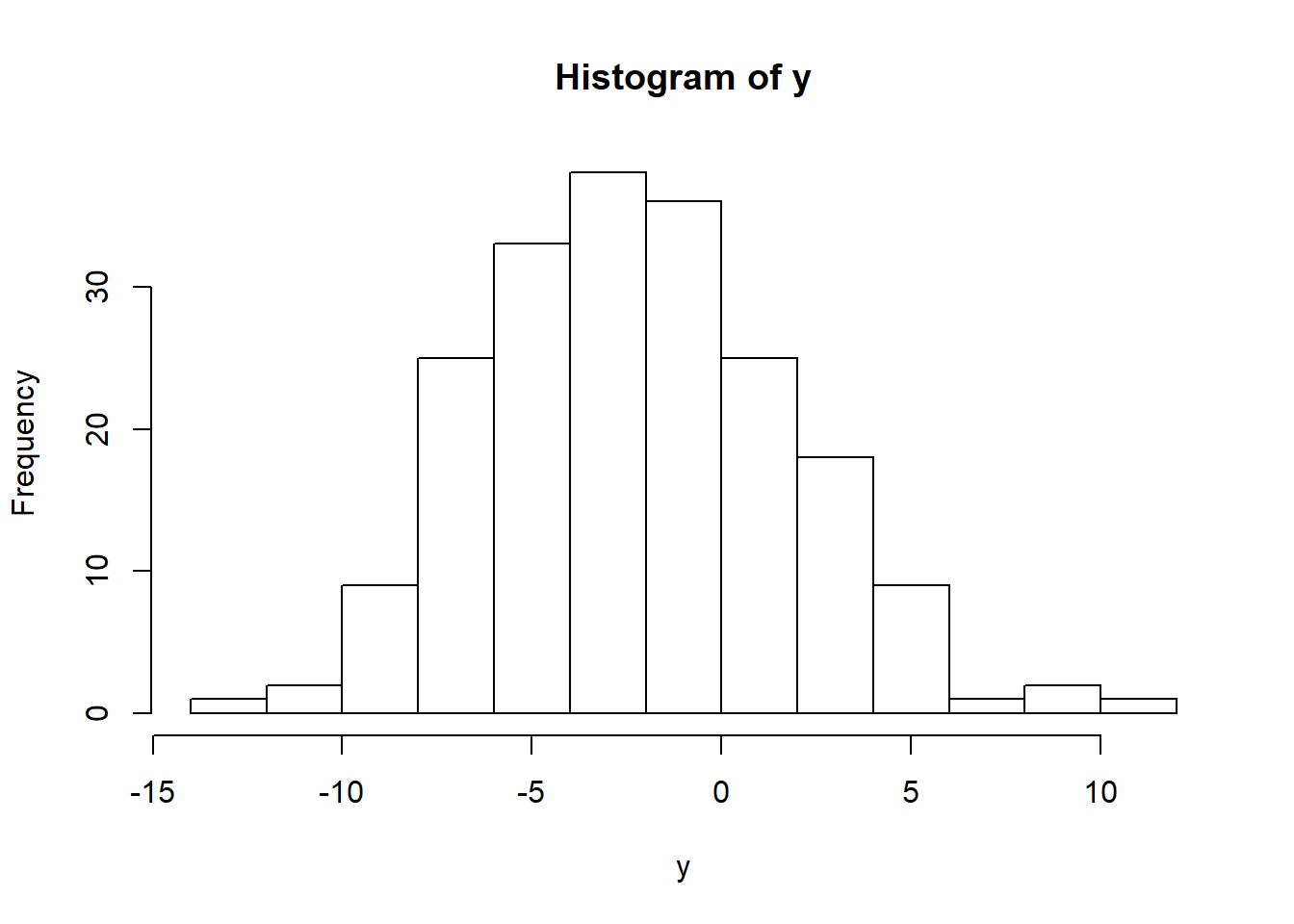``````qqnorm(y)
qqline(y)``````